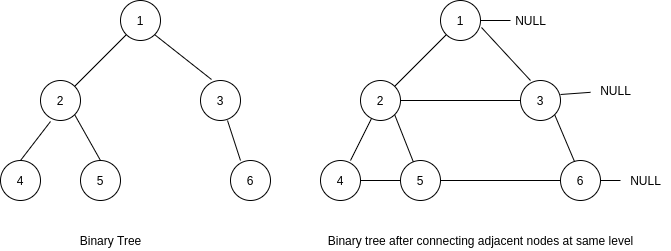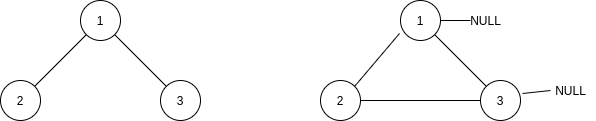Problem title
Difficulty
Avg time to solve

Min Heap
Moderate
30 mins
Maximum Subarray Sum
Moderate
25 mins
Minimum Cost to Destination
Hard
41 mins
Simplify the Directory
Moderate
22 mins
Search In Infinite Sorted 0-1 Array
Easy
10 mins
Minimize The Maximum
Easy
15 mins
Minimum Depth Of Binary Tree
Moderate
20 mins
Find Slope
Easy
22 mins
Minimum Number Of Lamps
Easy
10 mins
Kth largest element in the unsorted array
Moderate
10 mins7

# Connect Nodes at Same Level

Difficulty: MEDIUM
Avg. time to solve
30 min
Success Rate
70%

Problem Statement

#### You are given a binary tree, where the structure of the node is as follow -:

``````class BinaryTreeNode {
int data;      // Value of the node.
BinaryTreeNode *left;  // Pointer to left child node.
BinaryTreeNode *right; // Pointer to right child node.
BinaryTreeNode *next;  // Pointer to next right node at same level.
}
``````

#### For Example:

``````Consider the figure shown below. The left part represents the initial binary tree and right part represents the binary tree after connecting adjacent nodes at the same level.
````````````In the tree shown in the picture above -:
The ‘next’ pointer of the node having value 2 is connected to the node having value 3.
The ‘next’ pointer of the node having value 4 is connected to the node having value 5.
The ‘next’ pointer of the node having value 5 is connected to the node having value 6.
The ‘next’ pointer of nodes having value 1, 3, 6 will have a value NULL as there are no next right nodes in their cases.
``````

#### Note:

``````1. The structure of the ‘Node’ of a binary tree is already defined. You should not change it.
2. The root of the binary tree is known to you.
3. There is at least one node in the given binary tree.
4. You may only use constant extra space.
``````
##### Input format:
``````The first line of input contains an integer ‘T’ denoting the number of test cases.

Then the description of  ‘T’ test cases follows.

The first line of each test case contains elements in the level order form. The line consists of values of nodes separated by a single space. In case a node is null, we take -1 in its place.

For example, the input for the tree depicted in the below image would be :
````````````1
2 3
4 -1 5 6
-1 7 -1 -1 -1 -1
-1 -1
``````

#### Explanation :

``````Level 1 :
The root node of the tree is 1

Level 2 :
Left child of 1 = 2
Right child of 1 = 3

Level 3 :
Left child of 2 = 4
Right child of 2 = null (-1)
Left child of 3 = 5
Right child of 3 = 6

Level 4 :
Left child of 4 = null (-1)
Right child of 4 = 7
Left child of 5 = null (-1)
Right child of 5 = null (-1)
Left child of 6 = null (-1)
Right child of 6 = null (-1)

Level 5 :
Left child of 7 = null (-1)
Right child of 7 = null (-1)

The first not-null node (of the previous level) is treated as the parent of the first two nodes of the current level. The second not-null node (of the previous level) is treated as the parent node for the next two nodes of the current level and so on.
The input ends when all nodes at the last level are null (-1).
``````
##### Note :
``````The above format was just to provide clarity on how the input is formed for a given tree.
The sequence will be put together in a single line separated by a single space. Hence, for the above-depicted tree, the input will be given as:

1 2 3 4 -1 5 6 -1 7 -1 -1 -1 -1 -1 -1
``````
##### Output format :
``````For each test case, print the level order as connected by the next pointers, with '#' signifying the end of each level.
``````
##### Note:
``````You do not need to print anything, it has already been taken care of. Just implement the given function.
``````
##### Constraints:
``````1 <= T <= 100
1 <= N <= 3000
-10^9 <= DATA <= 10^9

Where ‘T’ is the total number of test cases, ‘N’ is the number of nodes in the given binary tree and 'DATA' is the value in each node.

Time Limit: 1 sec
``````
##### Sample Input 1:
``````1
1 2 3 -1 -1 -1 -1
``````
##### Sample Output 1:
``````1 # 2 3 #
``````
##### Explanation For Sample Input 1:
``````In the first test case, the level order traversal of a binary tree is 1, 2, 3, -1, -1,  thus its root is a node with value 1, and left and right child of the root are nodes with 2 and 3 respectively.
The initial binary tree and the binary tree after connecting adjacent nodes are shown below
The output is only for convenience,  It is in level order as connected by the next pointers, with '#' signifying the end of each level.
``````##### Sample Input 2:
``````1
1 2 3 4 5 -1 6 -1 -1 -1 -1 -1 -1
``````
##### Sample Output 2:
``````1 # 2 3 # 4 5 6 #
``````
##### Explanation For Sample Input 2:
``````In the first test case, see problem statement for its explanation.
``````Console